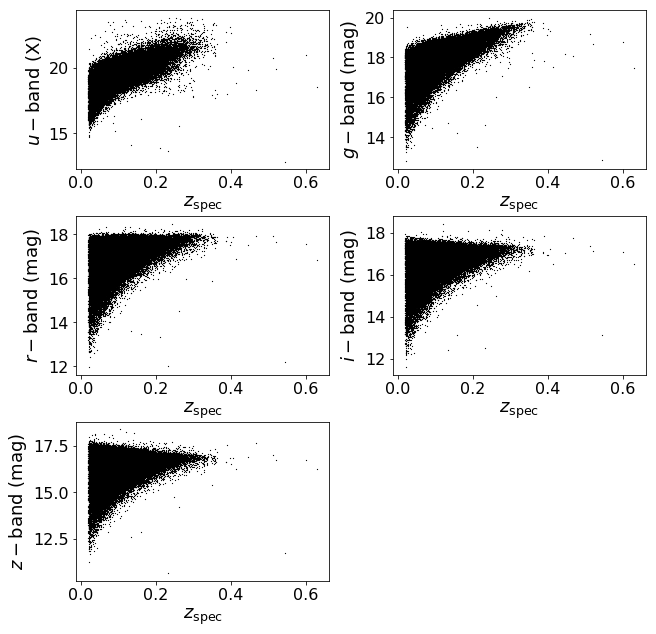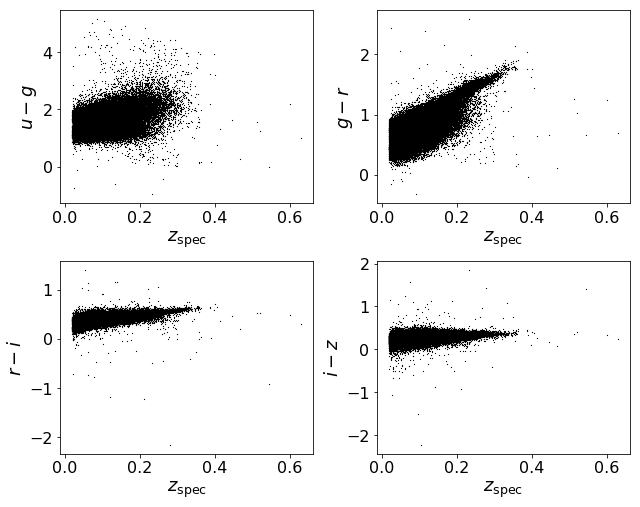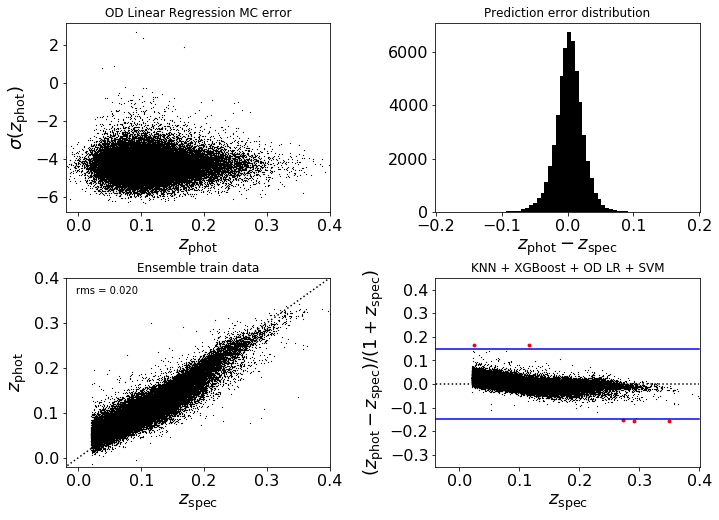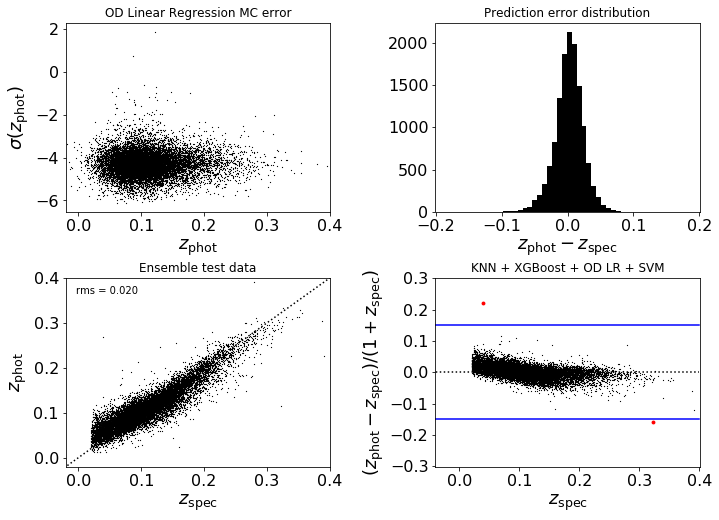## Photometric galaxy redshift determination using an Ensemble of Regressors¶

version 1 20/3/2018

Wing-Fai Thi

The ensemble includes k-Nearest Neighbors, XGBoost, Support Vector Machine, and an Orthogonal Distance Regression method based on ODRpack, which can account in the fit the errors for the predictor values.

The meta prediction is the weighted sum of the different predictions, where the weights are inversely proportional to the root-mean-square.

The expansion of the Universe makes that objects farther away from us are receding, resulting in a Doppler shift of their electromagnetic emission towards lower frequencies (hence redshift). wikipedia

Obtaining spectroscopic redshifts is a time-consuming endeavor compared to photometric redshifts, except that spectroscopic redshifts are much more precised and accurate. The challenge is to find the most precise and accurate photometric redshift determinations.

The data is described in Section 1.5.5 of Ref 1. The training set consists of u, g , r, i, z magnitudes and their associated erros of 60,000 galaxies from the SDSS spectroscopic sample from a total of ~600,000 galaxies.

Validation is performed on an additional 6000 galaxies. The left panel shows prediction error as a function of the spectroscopic redshift computed by Monte-Carlo simulation.

### Aim of the notebook¶

Apply different regressors and obtain the best possible estimations of the redshifts.

### Reference¶

1. "Statistics, Data Mining, and Machine Learning in Astronomy" (2013)

2. Analysis of a custom support vector machine for photometric redshift estimation and the inclusion of galaxy shape information E. Jones and J. Singal A&A 600, A113 (2017) preprint

3. Sloan Digital Sky Survey

4. Baysian approach photometric redshift determination by Nacisco Benitez (ApJ paper)

5. PHAT: PHoto-z Accuracy Testing a benchmark of different estimation methods. See the paper by Hildebrandt et al.

6. XGBoost package and the reference paper

The notebook is based on a Python script by Jake VanderPlas. For more information on the original code, see http://astroML.github.com. The original script has another definition of the rms.

In addition to the standard packages for data science (mumpy, matplotlib, sklearn), the notebook requires two additional packages (xgboost and astroML) as well as the ODLinear routines found on this website. The additional packages can be easily donwloaded (I use sudo pip install name_of_the_package) on a Mac.

In :
import numpy as np
import os # use for file reading/writing
import matplotlib as mpl
from matplotlib import pyplot as plt

from sklearn.model_selection import train_test_split
from sklearn.preprocessing import PolynomialFeatures
from sklearn.neighbors import KNeighborsRegressor
from sklearn.neural_network import MLPRegressor
from sklearn.ensemble import RandomForestRegressor
from sklearn.svm import SVR

from xgboost.sklearn import XGBRegressor # use the XGBoost routine
from ODLinear import OrthogonalDistanceLinearRegression

from astroML.datasets import fetch_sdss_specgals # routine to fetch the data from the web
from sklearn.externals import joblib

# get configuration current settings
#print (mpl.rcParams)

# Change the default settings
mpl.rcParams.update({'figure.figsize': (9.0, 7.0),
'figure.dpi' : 300, # 300 dpi for print
'font.size': 14,
'legend.frameon': False,
'legend.fontsize' : 12,
'xtick.labelsize' : 16,
'ytick.labelsize' : 16,
'axes.labelsize'  : 18
})

In :
#------------------------------------------------------------
# Fetch the data if it is not locally downloaded and prepare it for the computation
# I have it on a specific directory
#data = fetch_sdss_specgals()
data = fetch_sdss_specgals("/Users/Wing-Fai/Projects/astroML/astroML_data/")


We select only a fraction of the total available dataset and we remove a couple of outliers.

In :
# put magnitudes in a matrix
mag     = np.vstack([data['modelMag_%s' % f] for f in 'ugriz']).T
mag_err = np.vstack([data['modelMagErr_%s' % f] for f in 'ugriz']).T

# train/test on 10% of ~ 661 598 points
mag     = mag[::10]
mag_err = mag_err[::10]
z = data['z']
z = z[::10]

w = ((mag[:,1] < 20.) & (mag[:,2] < 18.5) & (mag[:,3] < 18.5) & (mag[:,4] < 18.5))
print "total number of objects:",mag.shape
print "number of objects retained:",mag[w].shape
mag = mag[w]
z   = z[w]

total number of objects: 66160
number of objects retained: 66091

In :
fig = plt.figure(figsize=(9, 9))
left=0.07, right=0.95,
bottom=0.07, top=0.95)

# u, g , r, i, z magnitudes
ax1.scatter(z,mag[:,0],s=1.5, lw=0, c='k')
ax1.set_xlabel(r'$z_{\rm spec}$')
ax1.set_ylabel(r'$u-{\rm band\ (X)}$')

ax1.scatter(z,mag[:,1],s=1.5, lw=0, c='k')
ax1.set_xlabel(r'$z_{\rm spec}$')
ax1.set_ylabel(r'$g-{\rm band\ (mag)}$')

ax1.scatter(z,mag[:,2],s=1.5, lw=0, c='k')
ax1.set_xlabel(r'$z_{\rm spec}$')
ax1.set_ylabel(r'$r-{\rm band\ (mag)}$')

ax1.scatter(z,mag[:,3],s=1.5, lw=0, c='k')
ax1.set_xlabel(r'$z_{\rm spec}$')
ax1.set_ylabel(r'$i-{\rm band\ (mag)}$')

ax1.scatter(z,mag[:,4],s=1.5, lw=0, c='k')
ax1.set_xlabel(r'$z_{\rm spec}$')
ax1.set_ylabel(r'$z-{\rm band\ (mag)}$')
fig.savefig('fig_photoz_magnitudes.png')
plt.show()The astronomical data are in magnitude scale, ie mag - mag_ref = -2.5 log10 (Flux/Flux_ref), where mag_ref and Flux_ref are the reference magnitude for a given reference flux. The primary flux reference is the star Vega, whose magnitudes are set to zero.

We can see the magnitude distribution in a flux (magnitude)-limited sky survey. As the flux decreases as 1/distance squared, the bright galaxies light are dimmed at high distances (ie at high redshifts). The faint galaxies high-redshift equivalent to the nearby galaxies cannot be detected.

We now create colors = magnitude(band 1) - magnitude(band 2). Notice that the Sloan survey did use unconventional photometric bands.

In :
# from the mangitudes u, g , r, i, z create the colors (u-g) (g-r) (r-i) (i-z)
col = np.empty(mag.shape)
col[:,0] = mag[:,0] - mag[:,1]
col[:,1] = mag[:,1] - mag[:,2]
col[:,2] = mag[:,2] - mag[:,3]
col[:,3] = mag[:,3] - mag[:,4]
col[:,4] = mag[:,0]

col_err = np.empty(mag_err.shape)
col_err[:,0] = np.sqrt(mag_err[:,0]**2 + mag_err[:,1]**2) # sig(1-2)= sqrt(sig1^1+sig2^2)
col_err[:,1] = np.sqrt(mag_err[:,1]**2 + mag_err[:,2]**2)
col_err[:,2] = np.sqrt(mag_err[:,2]**2 + mag_err[:,3]**2)
col_err[:,3] = np.sqrt(mag_err[:,3]**2 + mag_err[:,4]**2)
col_err[:,4] = mag_err[:,0]


Plot the colors.

In :
# plot the colors u-g, g-r , r-i, i-z
fig = plt.figure(figsize=(9, 7))
left=0.07, right=0.95,
bottom=0.07, top=0.95)
ax2.scatter(z,col[:,0],s=1.5, lw=0, c='k')
ax2.set_xlabel(r'$z_{\rm spec}$')
ax2.set_ylabel(r'$u-g$')

ax2.scatter(z,col[:,1],s=1.5, lw=0, c='k')
ax2.set_xlabel(r'$z_{\rm spec}$')
ax2.set_ylabel(r'$g-r$')

ax2.scatter(z,col[:,2],s=1.5, lw=0, c='k')
ax2.set_xlabel(r'$z_{\rm spec}$')
ax2.set_ylabel(r'$r-i$')

ax2.scatter(z,col[:,3],s=1.5, lw=0, c='k')
ax2.set_xlabel(r'$z_{\rm spec}$')
ax2.set_ylabel(r'$i-z$')
fig.savefig('fig_photoz_colors.png')
plt.show()We split the datasset into the trainning and test dataset. One can choose between trainning on the magnitudes or on the colors. The magnitudes (and colors) have measurement uncertainties.

In :
# splitting into 80%/20% train/test dataset
ind = np.arange(z.size)
ind_train, ind_test, z_train, z_test = train_test_split(ind, z,
test_size=0.2,random_state=2018)

if(True): # if True train on the colors
print "Train on the colors"
X_train = col[ind_train]
X_test  = col[ind_test]
X_train_err = col_err[ind_train]
X_test_err  = col_err[ind_test]
else:
print "Train on the magnitudes"
X_train = mag[ind_train]
X_test  = mag[ind_test]
X_train_err = mag_err[ind_train]
X_test_err  = mag_err[ind_test]

Train on the colors


We create here a dictionary with several regressors. One can modify the list to test your favorite routines.

• Extrem Gradient Boosting (XGBoost): a boosted Random Forest
• K Neighbors Regressor (KNN)
• Multi-layer Perceptron Neural Network (NN)
• Orthogonal Distance Linear Regression (ODLR) with 2nd degree polynomial features
• Non-linear Support Vector Machine Regressor (SVM), with RBF kernel
In :
#------------------------------------------------------------
# Compute the scores for several regressors

regressors = {'XGBoost': XGBRegressor(n_estimators=200,
max_depth=5,objective='reg:linear',
seed=2001,learning_rate=0.1),
'KNN': KNeighborsRegressor(15,weights='uniform'),
hidden_layer_sizes=(100,150,100),early_stopping=True),
'ODLR':OrthogonalDistanceLinearRegression(tol=1e-8),
'SVM': SVR(kernel='rbf', C=1e3, gamma=0.1)}

# Create polynomial features for the linear regressor
poly = PolynomialFeatures(degree=2,include_bias=False)
poly.fit(X_train)
X_poly_train = poly.transform(X_train)
X_poly_test  = poly.transform(X_test)
X_poly_train_err = poly.transform(X_train_err)
X_poly_test_err  = poly.transform(X_test_err)


In order of avoid training, one can used saved models. We use the joblib package from sklearn.

In :
filename = 'photoz_ensemble.sav'
regs  = []
if os.path.exists(filename): # read previously run models using joblib from sklearn

Read previously ran models


Run (train, predict) and save the regression models.

In :
names = []
test_preds = []
test_pred_errors = []
train_pred_errors = []
train_preds = []
train_scores = []
test_scores = []

for i, (name,regressor) in enumerate(regressors.items()):
print "Regressor ",name
if not (os.path.exists(filename)):
model = regressor
if(name == "ODLR"):
model.fit(X_poly_train, z_train, X_err = X_poly_train_err)
elif(name == "XGBoost"):
model.fit(X_train, z_train, early_stopping_rounds=10,
eval_set=[(X_test, z_test)], verbose=False)
else:
model.fit(X_train, z_train)
else:
model = regs[i]
# make predictions
if(name == "ODLR"):
y_pred = model.predict(X_poly_test)
train_pred = model.predict(X_poly_train)
test_pred_errors.append(model.predict_MC_error(X_poly_test,
X_err=X_poly_test_err,
Number_of_MC_iterations=100))
train_pred_errors.append(model.predict_MC_error(X_poly_train,
X_err=X_poly_train_err,
Number_of_MC_iterations=100))
train_score  = model.score(X_poly_train, z_train)
test_score  = model.score(X_poly_test, z_test)
else:
y_pred = model.predict(X_test)
train_pred = model.predict(X_train)
train_score  = model.score(X_train, z_train)
test_score  = model.score(X_test, z_test)
test_preds.append(y_pred)
train_preds.append(train_pred)
names.append(name)
regs.append(model)
train_scores.append(train_score)
test_scores.append(test_score)

# Save the model to disk
if not(os.path.exists(filename)):
print "Saving models"
joblib.dump(regs,filename)

Regressor  KNN
Regressor  ODLR
Regressor  SVM
Regressor  XGBoost
Regressor  NN


ODLR has a method to compute errors propgation from the observational uncertainties.

Here are the results from the training. Notice that special definition of rms used here (see Jones and Singal 2017)

In :
rms = []
print "Test score"
for (name,regressor),score,z_pred in zip(regressors.items(),test_scores,test_preds):
rms_regressor = np.sqrt((((z_pred-z_test)/(1+z_test))**2).sum()/z_test.size)
print name,(" - score: %1.3f" % score),(" - rms: %1.3f" % rms_regressor)
rms.append(rms_regressor)
rms = np.array(rms)

Test score
KNN  - score: 0.823  - rms: 0.020
ODLR  - score: 0.750  - rms: 0.023
SVM  - score: 0.500  - rms: 0.035
XGBoost  - score: 0.840  - rms: 0.019
NN  - score: 0.801  - rms: 0.021


Measurement errors can affect the prediction values. One simple way to estimate the error on the predictions is to perfom Monte-Carlo simulations.

In :
# Number_of_MC_iterations is the number of Monte-Carlo simulations
def predict_MC_error(X ,X_err, Number_of_MC_iterations=1000):
pred_MC=[]
for i in range(Number_of_MC_iterations) :
X_MC = np.random.normal(X, X_err)       # generate a random input
pred_MC.append(regressor.predict(X_MC)) # regressor is your regressor class instance
pred_MC = np.array(pred_MC)
std_pred = np.std(pred_MC,axis=0)
return std_pred

In :
regressor = regs
XGBoost_test_pred_errors = predict_MC_error(X_train ,X_train_err, Number_of_MC_iterations=100)
print("XGBoost prediction error propagation: %1.3f" %XGBoost_test_pred_errors.mean())

XGBoost prediction error propagation: 0.009

In :
# prediction errors based on Monte-Carlo simulation of galaxies with errors in magnitudes
# based on ODLR only
test_pred_errors = np.array(test_pred_errors).reshape(z_test.size)
train_pred_errors = np.array(train_pred_errors).reshape(z_train.size)
test_preds       = np.array(test_preds).T
train_preds = np.array(train_preds).T


The meta model (Ensemble) is simply the weighted mean between the different model predictions, with weight = 1/rms

In :
# the ensemble photometric redshift is just the mean between the three methods
# weighted mean with weight=1/rms
z_train_meta = (train_preds.dot(1./rms))/(1./rms).sum()
z_test_meta  = (test_preds.dot(1./rms))/(1./rms).sum()
rms_train_meta = np.sqrt((((z_train_meta-z_train)/(1+z_train))**2).sum()/z_train.size)
rms_meta       = np.sqrt((((z_test_meta-z_test)  / (1+z_test))**2).sum()/z_test.size)
print("meta train rms: %1.3f" % rms_train_meta)
print("meta test rms: %1.3f" % rms_meta)

meta train rms: 0.019
meta test rms: 0.020


Find the outliers in the predictions. The definition can be found in Jones and Singal (2017).

In :
# Outliers
woutliers_train = (abs(z_train_meta-z_train)/(1+z_train) > 0.15)
woutliers_test = (abs(z_test_meta-z_test)/(1+z_test) > 0.15)


Results for the training set. The outliers are shown in red inthe lower-right panel.

In :
fig = plt.figure(figsize=(10, 7))
left=0.07, right=0.95,
bottom=0.07, top=0.95)
#
j = np.argsort(z_train)
ax3.scatter(train_preds[:,1],np.log(train_pred_errors[j]),s=1.5, lw=0, c='k')
ax3.set_title("OD Linear Regression MC error")
ax3.set_ylabel(r'$\sigma(z_{\rm phot})$')
ax3.set_xlabel(r'$z_{\rm phot}$')
ax3.set_xlim(-0.02, 0.4001)
#
ax3.hist(z_train_meta-z_train, color='black', bins=100)
ax3.set_title("Prediction error distribution")
ax3.set_xlabel(r'$z_{\rm phot}-z_{\rm spec}$')
ax3.set_xlim(-0.2001, 0.2001)

#
ax3.scatter(z_train, z_train_meta, s=1.5, lw=0, c='k')
ax3.plot([-0.1, 0.4], [-0.1, 0.4], ':k')
ax3.text(0.04, 0.96, "rms = %.3f" % (rms_meta),
ha='left', va='top', transform=ax3.transAxes)

ax3.set_ylabel(r'$z_{\rm phot}$')
ax3.set_xlabel(r'$z_{\rm spec}$')

ax3.set_title("Ensemble train data")
ax3.set_xlim(-0.02, 0.4001)
ax3.set_ylim(-0.02, 0.4001)
ax3.xaxis.set_major_locator(plt.MultipleLocator(0.1))
ax3.yaxis.set_major_locator(plt.MultipleLocator(0.1))

ax3.scatter(z_train, (z_train_meta-z_train)/(1+z_train), s=1.5, lw=0, c='k')
z_train_outliers = z_train[woutliers_train]
z_train_meta_outliers = z_train_meta[woutliers_train]
ax3.scatter(z_train_outliers,
(z_train_meta_outliers-z_train_outliers)/(1+z_train_outliers), s=15, lw=0, c='red')

ax3.plot([-0.1, 0.4], [0.0, 0.0], ':k')
ax3.plot([-0.1, 0.4],[-0.15,-0.15], color='blue')
ax3.plot([-0.1, 0.4],[0.15,0.15], color='blue')

ax3.set_xlabel(r'$z_{\rm spec}$')
ax3.set_ylabel(r'$(z_{\rm phot}-z_{\rm spec})/(1+z_{\rm spec})$')

ax3.set_xlim(-0.04, 0.4001)
ax3.set_ylim(-0.3501, 0.4501)
ax3.set_title("KNN + XGBoost + OD LR + SVM")
ax3.xaxis.set_major_locator(plt.MultipleLocator(0.1))
ax3.yaxis.set_major_locator(plt.MultipleLocator(0.1))
fig.savefig('fig_photoz_Ensemble_train.png')The results for the test set.

In :
fig = plt.figure(figsize=(10, 7))
left=0.07, right=0.95,
bottom=0.07, top=0.95)
#
j = np.argsort(z_test)
ax4.scatter(test_preds[:,1],np.log(test_pred_errors[j]),s=1.5, lw=0, c='k')
ax4.set_title("OD Linear Regression MC error")
ax4.set_ylabel(r'$\sigma(z_{\rm phot})$')
ax4.set_xlabel(r'$z_{\rm phot}$')
ax4.set_xlim(-0.02, 0.4001)
#
ax4.hist(z_test_meta-z_test, color='black', bins=100)
ax4.set_title("Prediction error distribution")
ax4.set_xlabel(r'$z_{\rm phot}-z_{\rm spec}$')
ax4.set_xlim(-0.2001, 0.2001)

#
ax4.scatter(z_test, z_test_meta, s=1.5, lw=0, c='k')
ax4.plot([-0.1, 0.4], [-0.1, 0.4], ':k')
ax4.text(0.04, 0.96, "rms = %.3f" % (rms_meta),
ha='left', va='top', transform=ax4.transAxes)

ax4.set_ylabel(r'$z_{\rm phot}$')
ax4.set_xlabel(r'$z_{\rm spec}$')

ax4.set_title("Ensemble test data")
ax4.set_xlim(-0.02, 0.4001)
ax4.set_ylim(-0.02, 0.4001)
ax4.xaxis.set_major_locator(plt.MultipleLocator(0.1))
ax4.yaxis.set_major_locator(plt.MultipleLocator(0.1))

ax4.scatter(z_test, (z_test_meta-z_test)/(1+z_test), s=1.5, lw=0, c='k')
z_test_outliers = z_test[woutliers_test]
z_test_meta_outliers = z_test_meta[woutliers_test]
ax4.scatter(z_test_outliers,
(z_test_meta_outliers-z_test_outliers)/(1+z_test_outliers), s=15, lw=0, c='red')

ax4.plot([-0.1, 0.4], [0.0, 0.0], ':k')
ax4.plot([-0.1, 0.4],[-0.15,-0.15], color='blue')
ax4.plot([-0.1, 0.4],[0.15,0.15], color='blue')

ax4.set_xlabel(r'$z_{\rm spec}$')
ax4.set_ylabel(r'$(z_{\rm phot}-z_{\rm spec})/(1+z_{\rm spec})$')

ax4.set_xlim(-0.04, 0.4001)
ax4.set_ylim(-0.301, 0.301)
ax4.set_title("KNN + XGBoost + OD LR + SVM")
ax4.xaxis.set_major_locator(plt.MultipleLocator(0.1))
ax4.yaxis.set_major_locator(plt.MultipleLocator(0.1))

fig.savefig('fig_photoz_Ensemble_test.png')

plt.show()The ensemble of regressors manage a rms of 0.02 for both the training an the test datasets. The error due to the uncertainties in the magnitudes is much lower than the regression error (see upper-left panels). The prediction error distribution error is normal. One can see that the errors are positives for redshifts < 0.1. It does not make sense to have negative redshifts.

We employed a few of the most efficient regressors avaialble. The best-performing regressor is XGBoost (which is not surprising). The spread in errors is quite uniform as function of redshift. A scientist would prefer that the error being proportional to its value.

### Summary¶

In this notebook, we learn:

• how to change Matplotlib default settings
• how to use an ensemble of regressors
• a way to estimate the errors on the predictions due to measurement errors
• how to save and read machine learning models (sklearn.externals.joblib)
• simple astrononimal concepts like magnitude, color, adn redshift